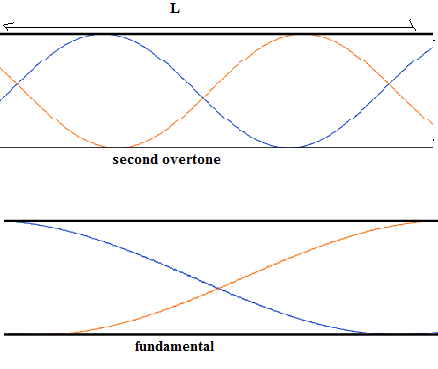QuestionAnswers

# The speed of sound in air is 333m/s. The fundamental frequency of the open pipe is 333Hz. The second overtone of the open organ pipe can be produced with a pipe of length:A. 0.5 mB. 1.0 mC. 1.5 mD. 2.0 m

Hint: Condition for production of standing waves is to be used here. Second overtone in an open pipe corresponds to a frequency that is around thrice the fundamental frequency. There are around three peaks of a wave that fit into the pipe in such a case.

Formula used:
The formula for the length of the pipe is:
$L = \dfrac{nv}{2 \nu}$
Where n= 1 for fundamental frequency and n= 3 for second overtone.In an open pipe, for fundamental frequency, to produce standing wave we require that the length of the pipe should be twice the wavelength of the fundamental mode or:
$\dfrac{\lambda}{2} = L$
The relation with frequency is:
$L = \dfrac{v}{2 \nu}$
where v is the velocity of sound in our case.
This is so because frequency and wavelength are related as:
$v=\nu \lambda$
For the second overtone, the wavelength is thrice of fundamental wavelength. Therefore, we replace $\lambda$ by $3 \times \lambda$

Keeping this in the formula for length and keeping the velocity of sound 333 m/s (given) in place of v:

$L = \dfrac{999 m/s}{2 \times 333 Hz}$
$L = \dfrac{ 3}{2}$m
L= 1.5 m

Therefore, the correct option is 1.5 m.

So, the correct answer is “Option C”.

The unit of frequency Hz is also $s^{-1}$ that is how we got cancellation of this in numerator and denominator and only m remained. Also the frequency and wavelength relation might get confusing. To sort it out, refer to the diagram; 3 times the new wavelength is twice the pipe length.